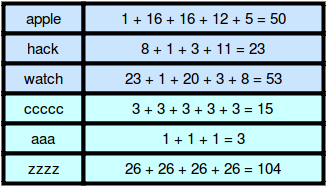# Weighted Uniform Strings in Algorithm | HackerRank Programming Solutions | HackerRank Problem Solving Solutions in Java [💯Correct]

Hello Programmers/Coders, Today we are going to share solutions of Programming problems of HackerRank, Algorithm Solutions of Problem Solving Section in Java. At Each Problem with Successful submission with all Test Cases Passed, you will get an score or marks. And after solving maximum problems, you will be getting stars. This will highlight your profile to the recruiters.

In this post, you will find the solution for Weighted Uniform Strings in Java-HackerRank Problem. We are providing the correct and tested solutions of coding problems present on HackerRank. If you are not able to solve any problem, then you can take help from our Blog/website.

`Use “Ctrl+F” To Find Any Questions Answer. & For Mobile User, You Just Need To Click On Three dots In Your Browser & You Will Get A “Find” Option There. Use These Option to Get Any Random Questions Answer.`

#### Introduction To Algorithm

The word Algorithm means “a process or set of rules to be followed in calculations or other problem-solving operations”. Therefore Algorithm refers to a set of rules/instructions that step-by-step define how a work is to be executed upon in order to get the expected results.

• It is easy to understand.
• Algorithm is a step-wise representation of a solution to a given problem.
• In Algorithm the problem is broken down into smaller pieces or steps hence, it is easier for the programmer to convert it into an actual program.

Link for the ProblemWeighted Uniform Strings– Hacker Rank Solution

`Weighted Uniform Strings – Hacker Rank Solution`

### Problem:

A weighted string is a string of lowercase English letters where each letter has a weight. Character weights are  to  from  to  as shown below:

• The weight of a string is the sum of the weights of its characters. For example:• uniform string consists of a single character repeated zero or more times. For example, `ccc` and `a` are uniform strings, but `bcb` and `cd` are not.

Given a string, , let  be the set of weights for all possible uniform contiguous substrings of string . There will be  queries to answer where each query consists of a single integer. Create a return array where for each query, the value is `Yes` if . Otherwise, append `No`.

Note: The  symbol denotes that  is an element of set .

Example

.

Working from left to right, weights that exist are:

```string  weight
a       1
b       2
bb      4
c       3
cc      6
ccc     9
d       4
dd      8
ddd     12
dddd    16
```

Now for each value in , see if it exists in the possible string weights. The return array is `['Yes', 'No', 'No', 'Yes', 'No']`.

Function Description

Complete the weightedUniformStrings function in the editor below.

weightedUniformStrings has the following parameter(s):
– string s: a string
– int queries[n]: an array of integers

Returns
– string[n]: an array of strings that answer the queries

Input Format

The first line contains a string , the original string.
The second line contains an integer , the number of queries.
Each of the next  lines contains an integer , the weight of a uniform subtring of  that may or may not exist.

Constraints

•  will only contain lowercase English letters, ascii[a-z].

Sample Input 0

```abccddde
6
1
3
12
5
9
10
```

Sample Output 0

```Yes
Yes
Yes
Yes
No
No
```

Explanation 0

The weights of every possible uniform substring in the string `abccddde` are shown below:

We print `Yes` on the first four lines because the first four queries match weights of uniform substrings of . We print `No` for the last two queries because there are no uniform substrings in  that have those weights.

Note that while `de` is a substring of  that would have a weight of , it is not a uniform substring.

Note that we are only dealing with contiguous substrings. So `ccc` is not a substring of the string `ccxxc`.

Sample Input 1

```aaabbbbcccddd
5
9
7
8
12
5
```

Sample Output 1

```Yes
No
Yes
Yes
No```
`Weighted Uniform Strings – Hacker Rank Solution`
```import java.io.*;
import java.util.*;
import java.text.*;
import java.math.*;
import java.util.regex.*;
public class Solution {
public static void main(String[] args) {
Scanner in = new Scanner(System.in);
String s = in.next();
int len =  s.length();
int n = in.nextInt();
Set<Integer> set = new HashSet<Integer>();
int i=0;
while(i<len){
int j=i;
int sum =0;
while( j<len && s.charAt(i)==s.charAt(j) ){
sum += (s.charAt(i)-'a') +1;
j++;
}
i = j;
}
for(int a0 = 0; a0 < n; a0++){
int x = in.nextInt();
if (set.contains(x)){
System.out.println("Yes");
}
else{
System.out.println("No");
}
}
}
}```